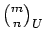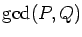Journal of Integer Sequences, Vol. 24 (2021), Article 21.1.4

## The p-Adic Valuation of Lucasnomials When p is a Special Prime

### Christian Ballot Normandie Université UNICAEN, CNRS Laboratoire de Mathématiques Nicolas Oresme 14000 Caen France

Abstract:

To determine the p-adic valuation of a Lucasnomial, i.e., of a generalized binomial coefficient with respect to a fundamental Lucas sequence U=U(P,Q), there is an adequate Kummer rule if p is a regular prime. No such rule exists if p is a special prime, i.e., if p divides. We provide a complete description of the p-adic valuation of Lucasnomials when p is special, with some numerical examples. Applications to the integrality of generalized Lucasnomial Fuss-Catalan numbers, Lucasnomial ballot and Lucasnomial Lobb numbers are also given.

Full version:  pdf,    dvi,    ps,    latex

Received July 3 2020; revised versions received December 12 2020; December 31 2020. Published in Journal of Integer Sequences, December 31 2020.

Return to Journal of Integer Sequences home page